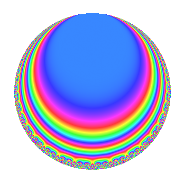Properties

 Label 128.3.d.cLevel 128 Weight 3 Character orbit 128.d Analytic conductor 3.488 Analytic rank 0 Dimension 4 CM No Inner twists 4

Related objects

Newspace parameters

 Level: $$N$$ = $$128 = 2^{7}$$ Weight: $$k$$ = $$3$$ Character orbit: $$[\chi]$$ = 128.d (of order $$2$$ and degree $$1$$)

Newform invariants

 Self dual: No Analytic conductor: $$3.48774738381$$ Analytic rank: $$0$$ Dimension: $$4$$ Coefficient field: $$\Q(\zeta_{8})$$ Coefficient ring: $$\Z[a_1, \ldots, a_{5}]$$ Coefficient ring index: $$2^{7}$$ Sato-Tate group: $\mathrm{SU}(2)[C_{2}]$

$q$-expansion

Coefficients of the $$q$$-expansion are expressed in terms of a basis $$1,\beta_1,\beta_2,\beta_3$$ for the coefficient ring described below. We also show the integral $$q$$-expansion of the trace form.

 $$f(q)$$ $$=$$ $$q$$ $$+ \beta_{2} q^{3}$$ $$+ \beta_{1} q^{5}$$ $$+ \beta_{3} q^{7}$$ $$- q^{9}$$ $$+O(q^{10})$$ $$q$$ $$+ \beta_{2} q^{3}$$ $$+ \beta_{1} q^{5}$$ $$+ \beta_{3} q^{7}$$ $$- q^{9}$$ $$+ 5 \beta_{2} q^{11}$$ $$-5 \beta_{1} q^{13}$$ $$+ \beta_{3} q^{15}$$ $$-10 q^{17}$$ $$+ 5 \beta_{2} q^{19}$$ $$+ 8 \beta_{1} q^{21}$$ $$-\beta_{3} q^{23}$$ $$+ 9 q^{25}$$ $$-10 \beta_{2} q^{27}$$ $$-5 \beta_{1} q^{29}$$ $$+ 40 q^{33}$$ $$-16 \beta_{2} q^{35}$$ $$+ 5 \beta_{1} q^{37}$$ $$-5 \beta_{3} q^{39}$$ $$-30 q^{41}$$ $$+ \beta_{2} q^{43}$$ $$-\beta_{1} q^{45}$$ $$-6 \beta_{3} q^{47}$$ $$-79 q^{49}$$ $$-10 \beta_{2} q^{51}$$ $$-15 \beta_{1} q^{53}$$ $$+ 5 \beta_{3} q^{55}$$ $$+ 40 q^{57}$$ $$-15 \beta_{2} q^{59}$$ $$+ 7 \beta_{1} q^{61}$$ $$-\beta_{3} q^{63}$$ $$+ 80 q^{65}$$ $$+ 29 \beta_{2} q^{67}$$ $$-8 \beta_{1} q^{69}$$ $$+ 5 \beta_{3} q^{71}$$ $$-10 q^{73}$$ $$+ 9 \beta_{2} q^{75}$$ $$+ 40 \beta_{1} q^{77}$$ $$+ 10 \beta_{3} q^{79}$$ $$-71 q^{81}$$ $$+ 9 \beta_{2} q^{83}$$ $$-10 \beta_{1} q^{85}$$ $$-5 \beta_{3} q^{87}$$ $$+ 22 q^{89}$$ $$+ 80 \beta_{2} q^{91}$$ $$+ 5 \beta_{3} q^{95}$$ $$+ 150 q^{97}$$ $$-5 \beta_{2} q^{99}$$ $$+O(q^{100})$$ $$\operatorname{Tr}(f)(q)$$ $$=$$ $$4q$$ $$\mathstrut -\mathstrut 4q^{9}$$ $$\mathstrut +\mathstrut O(q^{10})$$ $$4q$$ $$\mathstrut -\mathstrut 4q^{9}$$ $$\mathstrut -\mathstrut 40q^{17}$$ $$\mathstrut +\mathstrut 36q^{25}$$ $$\mathstrut +\mathstrut 160q^{33}$$ $$\mathstrut -\mathstrut 120q^{41}$$ $$\mathstrut -\mathstrut 316q^{49}$$ $$\mathstrut +\mathstrut 160q^{57}$$ $$\mathstrut +\mathstrut 320q^{65}$$ $$\mathstrut -\mathstrut 40q^{73}$$ $$\mathstrut -\mathstrut 284q^{81}$$ $$\mathstrut +\mathstrut 88q^{89}$$ $$\mathstrut +\mathstrut 600q^{97}$$ $$\mathstrut +\mathstrut O(q^{100})$$

Basis of coefficient ring:

 $$\beta_{0}$$ $$=$$ $$1$$ $$\beta_{1}$$ $$=$$ $$4 \zeta_{8}^{2}$$ $$\beta_{2}$$ $$=$$ $$-2 \zeta_{8}^{3} + 2 \zeta_{8}$$ $$\beta_{3}$$ $$=$$ $$8 \zeta_{8}^{3} + 8 \zeta_{8}$$
 $$1$$ $$=$$ $$\beta_0$$ $$\zeta_{8}$$ $$=$$ $$($$$$\beta_{3}\mathstrut +\mathstrut$$ $$4$$ $$\beta_{2}$$$$)/16$$ $$\zeta_{8}^{2}$$ $$=$$ $$\beta_{1}$$$$/4$$ $$\zeta_{8}^{3}$$ $$=$$ $$($$$$\beta_{3}\mathstrut -\mathstrut$$ $$4$$ $$\beta_{2}$$$$)/16$$

Character Values

We give the values of $$\chi$$ on generators for $$\left(\mathbb{Z}/128\mathbb{Z}\right)^\times$$.

 $$n$$ $$5$$ $$127$$ $$\chi(n)$$ $$-1$$ $$-1$$

Embeddings

For each embedding $$\iota_m$$ of the coefficient field, the values $$\iota_m(a_n)$$ are shown below.

For more information on an embedded modular form you can click on its label.

Label $$\iota_m(\nu)$$ $$a_{2}$$ $$a_{3}$$ $$a_{4}$$ $$a_{5}$$ $$a_{6}$$ $$a_{7}$$ $$a_{8}$$ $$a_{9}$$ $$a_{10}$$
63.1
 −0.707107 + 0.707107i −0.707107 − 0.707107i 0.707107 − 0.707107i 0.707107 + 0.707107i
0 −2.82843 0 4.00000i 0 11.3137i 0 −1.00000 0
63.2 0 −2.82843 0 4.00000i 0 11.3137i 0 −1.00000 0
63.3 0 2.82843 0 4.00000i 0 11.3137i 0 −1.00000 0
63.4 0 2.82843 0 4.00000i 0 11.3137i 0 −1.00000 0
 $$n$$: e.g. 2-40 or 990-1000 Significant digits: Format: Complex embeddings Normalized embeddings Satake parameters Satake angles

Inner twists

Char. orbit Parity Mult. Self Twist Proved
1.a Even 1 trivial yes
4.b Odd 1 yes
8.b Even 1 yes
8.d Odd 1 yes

Hecke kernels

This newform can be constructed as the kernel of the linear operator $$T_{3}^{2}$$ $$\mathstrut -\mathstrut 8$$ acting on $$S_{3}^{\mathrm{new}}(128, [\chi])$$.